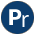#terms.pl -- Term manipulation

Compatibility library for term manipulation predicates. Most predicates in this library are provided as SWI-Prolog built-ins.

Compatibility
- YAP, SICStus, Quintus. Not all versions of this library define exactly the same set of predicates, but defined predicates are compatible.
term_size(@Term, -Size) is det
True if Size is the size in cells occupied by Term on the global (term) stack. A cell is 4 bytes on 32-bit machines and 8 bytes on 64-bit machines. The calculation does take sharing into account. For example:
```?- A = a(1,2,3), term_size(A,S).
S = 4.
?- A = a(1,2,3), term_size(a(A,A),S).
S = 7.
?- term_size(a(a(1,2,3), a(1,2,3)), S).
S = 11.```

Note that small objects such as atoms and small integers have a size 0. Space is allocated for floats, large integers, strings and compound terms.

variant(@Term1, @Term2) is semidet
Same as SWI-Prolog `Term1 =@= Term2`.
subsumes_chk(@Generic, @Specific)
True if Generic can be made equivalent to Specific without changing Specific.
deprecated
- Replace by subsumes_term/2.
subsumes(+Generic, @Specific)
True if Generic is unified to Specific without changing Specific.
deprecated
- It turns out that calls to this predicate almost always should have used subsumes_term/2. Also the name is misleading. In case this is really needed, one is adviced to follow subsumes_term/2 with an explicit unification.
term_subsumer(+Special1, +Special2, -General) is det
General is the most specific term that is a generalisation of Special1 and Special2. The implementation can handle cyclic terms.
author
- Inspired by LOGIC.PRO by Stephen Muggleton
Compatibility
- SICStus
lgg_safe(+S1, +S2, -G, +Map0, -Map) is det[private]
Cycle-safe version of the above. The difference is that we insert compounds into the mapping table and check the mapping table before going into a compound.
term_factorized(+Term, -Skeleton, -Substiution)
Is true when Skeleton is Term where all subterms that appear multiple times are replaced by a variable and Substitution is a list of Var=Value that provides the subterm at the location Var. I.e., After unifying all substitutions in Substiutions, Term == Skeleton. Term may be cyclic. For example:
```?- X = a(X), term_factorized(b(X,X), Y, S).
Y = b(_G255, _G255),
S = [_G255=a(_G255)].```

## Undocumented predicates

The following predicates are exported, but not or incorrectly documented.

term_hash(Arg1, Arg2, Arg3, Arg4)
term_hash(Arg1, Arg2)
term_variables(Arg1, Arg2, Arg3)
cyclic_term(Arg1)